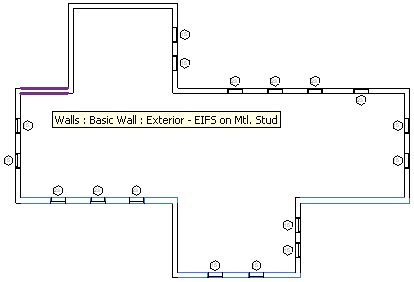### South Facing Walls

This is part 3 of Scott Conover's AU 2009 class on analysing building geometry, demonstrating how to find and highlight south facing exterior walls.

The previous post discussed curve parametrisation. In this step, we use the tangent vector to the wall location curve to find all exterior walls in the model facing south:The code we discuss is for demonstration purposes on how to use the curve properties. This sample demonstrates the analysis required to determine the facing direction of a wall, which has a location curve. For the sake of brevity, we will not show all the code here, only relevant snippets. The complete solution is available in Scott's AU class materials.

In order to highlight the exterior walls facing south, we need to perform the following steps:

• Collect all exterior walls.
• For each wall, determine its facing direction.
• If it is south facing, select it.

These three steps are implemented in the following methods:

• CollectExteriorWalls, which finds all exterior walls in the active document and returns an enumerable containing them.
• GetExteriorWallDirection to obtain the outward direction of an exterior wall.
• The mainline code handling the selection and highlighting.

Here is the implementation of CollectExteriorWalls, which also shows how to make use of a LINQ query to filter out the exterior walls and create an IEnumerable collection:

```protected IEnumerable<Wall> CollectExteriorWalls()
{
List<Element> elementsToProcess
= new List<Element>();

Autodesk.Revit.Creation.Filter cf
= Application.Create.Filter;

TypeFilter wallFilter = cf.NewTypeFilter(
typeof( Wall ), true );

Document.get_Elements( wallFilter, elementsToProcess );

// Use a LINQ query to filter out only exterior walls

IEnumerable<Wall> exteriorWalls
= from wall in elementsToProcess.Cast<Wall>()
where IsExterior( wall.ObjectType )
select wall;

return exteriorWalls;
}
```

The LINQ query uses a predicate method IsExterior which checks the wall built-in FUNCTION_PARAM parameter value to determine whether a wall is exterior or not:

```protected bool IsExterior( Symbol wallType )
{
Parameter wallFunction = wallType.get_Parameter(
BuiltInParameter.FUNCTION_PARAM );

WallFunction value
= ( WallFunction ) wallFunction.AsInteger();

return WallFunction.Exterior == value;
}
```

To determine the wall facing direction, we first we determine the direction of the wall curve itself, differentiating between straight and curved walls. For a straight wall, we call the ComputeDerivatives method on the location curve, which is a straight line, and use its BasisX vector, which is the tangent vector or the first derivative. For a curved wall, we simply compute the direction from its start to end point. This calculation will yield the same result as ComputeDerivatives for a straight line as well, so this differentiation could actually be skipped.

The direction facing outward is the normal vector of the wall curve direction, which can be obtained by forming the cross product with the Z axis, assuming that the wall is vertical.

We also need to check the wall's Flipped property. If set, the exterior direction is reversed. This is the resulting algorithm:

```protected XYZ GetExteriorWallDirection( Wall wall )
{
LocationCurve locationCurve
= wall.Location as LocationCurve;

XYZ exteriorDirection = XYZ.BasisZ;

if( locationCurve != null )
{
Curve curve = locationCurve.Curve;

//Write("Wall line endpoints: ", curve);

XYZ direction = XYZ.BasisX;

if( curve is Line )
{
// Obtains the tangent vector of the wall.

direction = curve.ComputeDerivatives(
0, true ).BasisX.Normalized;
}
else
{
// An assumption, for non-linear walls,
// that the "tangent vector" is the direction
// from the start of the wall to the end.

direction = ( curve.get_EndPoint( 1 )
- curve.get_EndPoint( 0 ) ).Normalized;
}

// Calculate the normal vector via cross product.

exteriorDirection = XYZ.BasisZ.Cross( direction );

// Flipped walls need to reverse the calculated direction

if( wall.Flipped )
{
exteriorDirection = -exteriorDirection;
}
}
return exteriorDirection;
}
```

The south facing walls are defined to be all those whose exterior direction is within a range of -45 degrees to 45 degrees to the south vector, the negative Y axis:

```protected bool IsSouthFacing( XYZ direction )
{
double angleToSouth = direction.Angle(
-XYZ.BasisY );

return Math.Abs( angleToSouth ) < Math.PI / 4;
}
```

Here is the mainline putting all of this together and adding some code to place the resulting walls in the document selection set, thus causing them to be highlighted on the graphics screen:

```SelElementSet selElements = Document.Selection.Elements;

IEnumerable<Wall> walls = CollectExteriorWalls();

foreach( Wall wall in walls )
{
XYZ exteriorDirection = GetExteriorWallDirection( wall );

bool isSouthFacing = IsSouthFacing( exteriorDirection );

if( isSouthFacing )
{